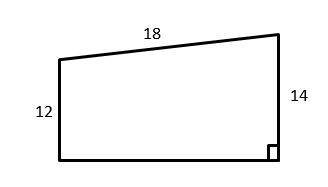# Top 10 5th Grade Common Core Math Practice QuestionsThe best way to prepare your students for the 5th grade Common Core Math test is to work through as many 5th Grade Common Core Math practice questions as possible. Here are the top 10 5th grade Common Core Math practice questions to help students review the most important 5th grade Common Core Math concepts. These 5th grades Common Core Math practice questions are designed to cover mathematics concepts and topics that are found on the actual test. The questions have been fully updated to reflect the latest 2021 5th grade Common Core guidelines. Answers and full explanations are provided at the end of the post.

Let your students start their Common Core Math test prep journey right now with these sample 5th grade Common Core Math questions.

## 5th grade Common Core Math Practice Questions

1- In a bag, there are 65 cards. Of these cards, 13 cards are white. What fraction of the cards are white?

A. $$\frac{1}{5}$$

B. $$\frac{2}{7}$$

C. $$\frac{13}{52}$$

D. $$\frac{1}{4}$$

2- Which expression is equal to $$\frac{3}{11}$$ ?

A. $$3 – 11$$

B. $$3 ÷ 11$$

C. $$3 × 11$$

D. $$\frac{11}{3}$$

3- If $$A = 20$$, then which of the following equations are correct?

A. $$A + 20 = 40$$

B. $$A ÷ 20 = 40$$

C. $$20 × A = 40$$

D. $$A – 20 = 40$$

4- How long does a 425 miles trip take moving at 50 miles per hour (mph)?

A. 4 hours

B. 6 hours and 24 minutes

C. 8 hours and 24 minutes

D. 8 hours and 30 minutes

5- 50 is What percent of 40?

A. $$20 \%$$

B. $$25 \%$$

C. $$125 \%$$

D. $$150 \%$$

6- The perimeter of the trapezoid below is 52. What is its area? _________7- The area of a circle is $$64π$$. What is the circumference of the circle?

A. $$8 π$$

B. $$16 π$$

C. $$32 π$$

D. $$64 π$$

8- A rope weighs 800 grams per meter of length. What is the weight in kilograms of 12.2 meters of this rope? (1 kilograms = 1000 grams)

A. 0.0976

B. 0.976

C. 9.76

D. 9760

9- Solve.
$$\frac{1}{2} + \frac{4}{5} – \frac{3}{10} = ?$$

A. $$\frac{9}{10}$$

B. $$\frac{2}{10}$$

C. 1

D. 2

10- How many $$\frac{1}{4}$$ cup servings are in a package of cheese that contains $$6 \frac{1}{2}$$ cups altogether?_________

## Answers:

1- A
There are 65 cards in the bag and 13 of them are white. Then, 13 out of 65 cards are white. You can write this as: $$\frac{13}{65}$$.
To simplify this fraction, divide both numerator and denominator by 13. Then:
$$\frac{13}{65}=\frac{1}{5}$$

2- B
$$\frac{3}{11}$$ means 3 is divided by 11. The fraction line simply means division or $$÷$$. Therefore, we can write $$\frac{3}{11}$$ as $$3 ÷ 11$$.

3- A
Plug in 20 for A in the equations. Only option A works.
$$A+20=40$$
$$20+20=40$$

4- D
50 miles : 1 hour
425 miles : $$425 ÷ 50 = 8.5$$ hours

5- C
Use percent formula:
$$part =\frac{percent}{100}× whole$$
$$50=\frac{percent}{100}× 40⇒ 50 = \frac{percent ×40}{100}⇒ 50 = \frac{percent ×4}{10}$$
multiply both sides by 10.
500 = percent $$×4$$, divide both sides by 4.
125 = percent

6- 104
First, find the missing side of the trapezoid. The perimeter of the trapezoid below is 52. Therefore, the missing side of the trapezoid (its height) is: $$52-12-18-14=52-44=8$$
Area of a trapezoid: $$A = \frac{1}{2} h (b_{1} + b_{2}) = \frac{1}{2} (8) (12 + 14) = 104$$

7- B
Use area and circumference of circle formula.
Area of a circle = $$πr^2 ⇒ 64π = πr^2 ⇒ r= 8$$
Circumference of a circle = $$2πr ⇒ C = 2×8×π ⇒ C =16π$$

8- C
1 meter of the rope = 800 grams 12.2 meter of the rope = $$12.2 × 800 =9,760$$grams = 9.76 kilograms

9- C
$$\frac{1}{2} + \frac{4}{5} – \frac{3}{10} = \frac{(5×1)+(2×4)-(1×3)}{10} =\frac{10}{10}=1$$

10- 26
To solve this problem, divide $$6\frac{1}{2}$$ by $$\frac{1}{4}$$.
$$6\frac{1}{2}÷\frac{1}{4}=\frac{13}{2}÷\frac{1}{4}=\frac{13}{2}×\frac{4}{1}=26$$

Looking for the best resource to help you succeed on the 5th grade Common Core Math test?

### The Best Books to Ace the 5th grade Common Core Math Test30% OFF

X

## How Does It Work?### 1. Find eBooks

Locate the eBook you wish to purchase by searching for the test or title.### 2. Add to Cart

Add the eBook to your cart.### 3. Checkout

Complete the quick and easy checkout process.### 4. Download

Immediately receive the download link and get the eBook in PDF format.

## Why Buy eBook From Effortlessmath?Save up to 70% compared to printInstantly download and access your eBookHelp save the environmentLifetime access to your eBookOver 2,000 Test Prep titles availableOver 80,000 happy customersOver 10,000 reviews with an average rating of 4.5 out of 524/7 supportAnytime, Anywhere Access